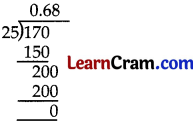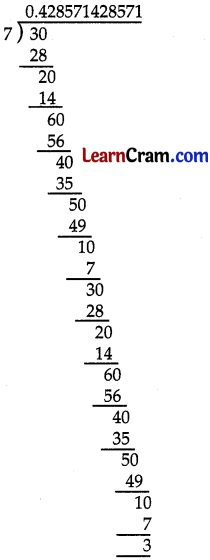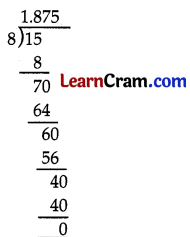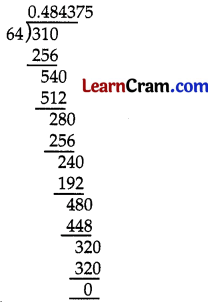# DAV Class 7 Maths Chapter 3 Worksheet 1 Solutions

The DAV Class 7 Maths Book Solutions Pdf and DAV Class 7 Maths Chapter 3 Worksheet 1 Solutions of Rational Numbers as Decimals offer comprehensive answers to textbook questions.

## DAV Class 7 Maths Ch 3 WS 1 Solutions

Question 1.
Express the following rational numbers as decimals:
(i) $$\frac{9}{8}$$
$$\frac{9}{8}=\frac{9 \times 125}{8 \times 125}$$
= $$\frac{1125}{1000}$$
= 1.125

(ii) $$\frac{615}{125}$$
$$\frac{615}{125}=\frac{123}{25}=\frac{123 \times 4}{25 \times 4}$$
= $$\frac{492}{100}$$
= 4.92(iii) $$\frac{1}{16}$$
$$\frac{1}{16}=\frac{1 \times 625}{16 \times 625}$$
= $$\frac{-75}{100}$$
= 0.0625

(iv) $$\frac{-3}{4}$$
$$\frac{-3}{4}=\frac{-3 \times 25}{4 \times 25}$$
= $$\frac{295}{1000}$$
= -0.75

(v) $$\frac{59}{200}$$
$$\frac{59}{200}=\frac{59 \times 5}{200 \times 5}$$
= $$\frac{295}{1000}$$
= 0.295

(vi) $$\frac{-24}{25}$$
$$\frac{-24}{25}=\frac{-24 \times 4}{25 \times 4}$$
= $$\frac{-96}{100}$$
= -0.96(vii) $$\frac{-53}{250}$$
$$\frac{-53}{250}=\frac{-53 \times 4}{250 \times 4}$$
= $$\frac{-212}{1000}$$
=-0.212

(viii) $$\frac{47}{400}$$
$$\frac{47}{400}=\frac{47 \times 25}{400 \times 25}$$
= $$\frac{1175}{10000}$$
= 0.1175(ix) $$\frac{27}{800}$$
$$\frac{27}{800}=\frac{27 \times 125}{800 \times 125}$$
= $$\frac{3375}{100000}$$
= 0.03375

(x) $$\frac{139}{625}$$
$$\frac{139}{625}=\frac{139 \times 16}{625 \times 16}$$
= $$\frac{2224}{10000}$$
=0.2224

(xi) $$\frac{3186}{1250}$$
$$\frac{3186}{1250}=\frac{3186 \times 8}{1250 \times 8}$$
= $$\frac{25488}{10000}$$
= 2.5488

(xii) $$\frac{133}{25}$$
$$\frac{133}{25}=\frac{133 \times 4}{25 \times 4}$$
= $$\frac{532}{100}$$
= 5.32### DAV Class 8 Maths Chapter 3 Worksheet 1 Notes

Conversion of a rational number into decimal form whose denominator is not 10 or a power of 10.
e.g. $$\frac{3}{5}=\frac{3 \times 2}{5 \times 2}$$
$$\frac{6}{10}$$ = 0.6

Rational numbers whose denominators can not be converted into 10 or a power of 10 are represented in the form of decimal by using long division method. Such representations may be either terminating or non-terminating but repeating.

Terminating Decimals:
The rational numbers whose denominators are 2 or 5 as their prime factors are represented in the form of terminating decimal system.
e.g. $$\frac{13}{125}, \frac{26}{100}, \frac{37}{80}$$ etc.

Non-terminating Decimals:
The rational numbers whose denominators are not 2 or 5 as their prime factors, are represented in the form of non-terminating decimal system using Long division method.
e.g. $$\frac{25}{36}, \frac{7}{3}, \frac{2}{7}$$

Non-terminating repeating decimal numbers:
Decimal numbers having an infinite number of decimal places and a set of digits in the decimal places that repeat are known as non- terminating repeating decimal numbers.

For example, 0.286715………… = $$0 . \overline{286715}$$ is non-terminating repeating decimal number. After 5, the same digits, i.e., 2, 8, 6, 7, 1, 5 will keep on repeating again arid again.

To convert a terminating decimal into rational number perform the following steps:

• Count the number of decimal places in the given number.
• Write the given number without the decimal point.
• The denominator is one followed by as many zeroes as is the number of decimal places in the given number.Example 1:
Convert $$\frac{3}{5}$$ in decimal form.
Here, the denominator is 5
∴ $$\frac{3}{5}=\frac{3 \times 2}{5 \times 2}$$
= $$\frac{6}{10}$$ = 0.6

Example 2:
Represent the following rational numbers as decimals using division method.
(i) $$\frac{3}{8}$$∴ $$\frac{3}{8}$$ = 0.375

(ii) $$\frac{17}{25}$$∴ $$\frac{17}{25}$$ = 0.68

(iii) $$\frac{124}{40}$$∴ $$\frac{124}{40}$$ = 3.1Example 3:
Convert the following rational numbers in decimal form.

(i) $$\frac{3}{7}$$∴ $$\frac{3}{7}$$ = 0.428571428571
= $$0 . \overline{428571}$$ (Non-terminating and repeating decimal)

(ii) $$\frac{125}{3}$$∴ $$\frac{125}{3}$$ = 41.666…………
= $$41 . \overline{6}$$ (Non-terminating and repeating decimal)

(iii) $$\frac{1025}{24}$$∴ $$\frac{1025}{24}$$ = 42.7083333….
= $$42.708 \overline{3}$$ (Non-terminating and repeating decimal)Example 4:
Without actual division, convert the following rational numbers into terminating decimals.

(i) $$\frac{45}{24}$$
24 = 2 × 2 × 2 × 3
Prime factors of 24 are not 2 and 5 but is not in its lowest form.
$$\frac{45}{24}=\frac{15 \times 3}{8 \times 3}=\frac{15}{8}$$
The prime factors of 8 are 2 × 2 × 2∴ $$\frac{45}{24}$$ = $$\frac{15}{8}$$ = 1.875

(ii) $$\frac{31}{64}$$∴ $$\frac{31}{64}$$ = 0.484375 (Terminating decimal)